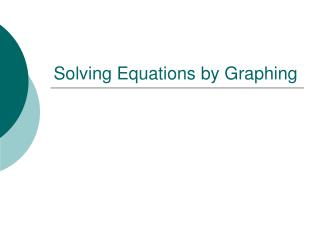DownloadDownload PresentationSolving Equations by Graphing

# Solving Equations by Graphing

Download Presentation## Solving Equations by Graphing

- - - - - - - - - - - - - - - - - - - - - - - - - - - E N D - - - - - - - - - - - - - - - - - - - - - - - - - - -
##### Presentation Transcript

1. Solving Equations by Graphing

2. Solving Equations • Solving an equation means finding the value of x if y= 0 • There are several ways to solve equations but we are going to focus on graphing, factoring, and the quadratic formula

3. Solving Equations by Graphing • If you look at a graph, where does y ALWAYS equal 0? • The x-axis • To solve an equation by graphing, determine where the graph crosses the x-axis. • What names do we have for this point: x-intercept, root, zero, solution

4. Solve: y= |x+ 3| - 4 • What type of equation? • Absolute value • What does it mean to ‘solve’ it? • Find the value of xwhen y = 0. • How could we do this by hand? • 0 = |x + 3| - 4

5. 0 = |x + 3| - 4 Isolate the absolute value expression. 4 = |x + 3| Split into two equation and solve 4 = x+ 3 -4 = x+ 3 1 = x-7 =x

6. Solve: y = |x + 3| - 4 • When we solve by hand, we determine that x = 1, -7 • Graph y = |x + 3| - 4 and determine the zeros. Where does the graph cross the x-axis? x = 1, -7

7. What are the solutions to the equations represented below? What type of function is this? What type of function is this? x = -3, 0, 4 x = -5, 1 What are the solutions to this cubic function? What are the solutions to this quadratic function?

8. Find the solution to y = 2x - 1 • What type of equation is it? • Exponential • x = 0

9. Find the roots of y = 3x2 + x - 2 What type of equation is this? Quadratic x = -1 x = ? What are the roots?

10. Find the roots of y = 3x2 + x - 2 • -1 is obviously a solution, but it is hard to determine the other solution. • The calculator will determine it for you: • Graph the function • Hit: G-Solv (F5) then Root (F1) (notice it gives the xand yvalues, what is the yvalue??) • x = .6666667 is 2/3(use the fraction not the decimal!!!)

11. Find the roots of y = 3x2 + x - 2 • The roots of y = 3x2 + x – 2 are x= -1, 2/3

12. Find the roots of y = x2+ 1 • What type of equation is it? • Quadratic • What does it mean to find the ‘roots’? • Find the value of x when y = 0. • How could we do this by hand? • 0 = x2 + 1 • -1 = x2 • ±i = x • What does this mean?? Let’s look at the graph.

13. Find the roots of y = x2+ 1 • It doesn’t cross the x =axis. So our solution is… • x = ø

14. Name the type of function and find the zeros using the calculator. zeros x = 1.2619 Exponential x = 0, -7 Cubic x = 2.5 Square Root